# Sea Clutter Simulation for a Maritime Radar System

This example will introduce a sea clutter simulation for a maritime surveillance radar system. This example will first discuss the physical properties associated with sea states. Next, we will discuss the reflectivity of sea surfaces, investigating the effect of sea state, frequency, polarization, and grazing angle. Lastly, we will calculate the clutter-to-noise ratio (CNR) for a maritime surveillance radar system, considering the propagation path and weather effects.

### Overview of Sea States

In describing sea clutter, it is first important to establish the physical properties of the sea surface. In modeling sea clutter for radar, there are 3 important parameters:

1. ${\sigma }_{\mathit{h}}$ is the standard deviation of the wave height. The wave height is defined as the vertical distance between the wave crest and the adjacent wave trough.

2. ${\beta }_{0\text{\hspace{0.17em}}}$is the slope of the wave.

3. ${\mathit{v}}_{\mathit{w}\text{\hspace{0.17em}}}$is the wind speed.

Due to the irregularity of waves, the physical properties of the sea are often described in terms of sea states. The Douglas sea state number is a widely used scale that represents a wide range of physical sea properties such as wave heights and associated wind velocities. At the lower end of the scale, a sea state of 0 represents a calm, glassy sea state. The scale then proceeds through states that represent everything from slightly rippled at 1 to rough seas with high wave heights at sea state 5. Wave heights at a sea state of 8 can be greater than 9 m or more.

Using the searoughness function, plot the sea properties for sea states 1 through 5. Note the slow increase in the wave slope ${\beta }_{0\text{\hspace{0.17em}}}$with sea state. This is a result of the wavelength and wave height increasing with wind speed, albeit with different factors.

% Analyze for sea states 1 through 5 ss = 1:5; % Sea states % Initialize outputs numSeaStates = numel(ss); hgtsd = zeros(1,numSeaStates); beta0 = zeros(1,numSeaStates); vw= zeros(1,numSeaStates); % Obtain sea state properties for is = 1:numSeaStates [hgtsd(is),beta0(is),vw(is)] = searoughness(ss(is)); end % Plot results helperPlotSeaRoughness(ss,hgtsd,beta0,vw);The physical properties introduced will be an important part in developing the geometry and environment of the maritime scenario. Furthermore, as we will see, radar returns from a sea surface exhibit strong dependence on sea state.

### Reflectivity

The sea surface is composed of water with an average salinity of about 35 parts per thousand. The reflection coefficient of sea water is close to -1 for microwave frequencies and at low grazing angles.

For smooth seas, the wave height is small, and the sea appears as an infinite, flat conductive plate with little-to-no backscatter. As the sea state number increases and the wave height increases, the surface roughness increases. This results in increased scattering that is directionally dependent. Additionally, the reflectivity exhibits a strong proportional dependence on wave height and a dependence that increases with increasing frequency on wind speed.

Investigate sea surface reflectivity versus frequency for various sea states using the seareflectivity function. Set the grazing angle equal to 0.5 degrees and consider frequencies over the range of 500 MHz to 35 GHz.

grazAng = 0.5; % Grazing angle (deg) freq = linspace(0.5e9,35e9,100); % Frequency (Hz) pol = 'H'; % Horizontal polarization % Initialize reflectivity output numFreq = numel(freq); nrcsH = zeros(numFreq,numSeaStates); % Calculate reflectivity for is = 1:numSeaStates nrcsH(:,is) = seareflectivity(ss(is),grazAng,freq,'Polarization',pol); end % Plot reflectivity helperPlotSeaReflectivity(ss,grazAng,freq,nrcsH,pol);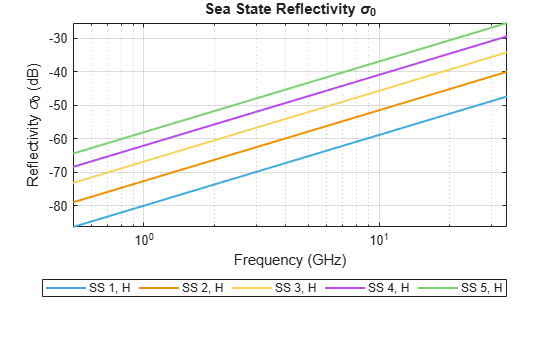As can be see from the figure above, the sea surface reflectivity is proportional to frequency. Additionally, as the sea state number increases, which corresponds to increasing roughness, the reflectivity also increases.

### Polarization Effects

Next consider polarization effects on the sea surface reflectivity. Maintain the same grazing angle and frequency span previously used.

pol = 'V'; % Vertical polarization % Initialize reflectivity output numFreq = numel(freq); nrcsV = zeros(numFreq,numSeaStates); % Calculate reflectivity for is = 1:numSeaStates nrcsV(:,is) = seareflectivity(ss(is),grazAng,freq,'Polarization',pol); end % Plot reflectivity hAxes = helperPlotSeaReflectivity(ss,grazAng,freq,nrcsH,'H'); helperPlotSeaReflectivity(ss,grazAng,freq,nrcsV,'V',hAxes);As can be seen from the figure above, there is a noticeable effect on the reflectivity based on polarization. Notice that the difference between horizontal and vertical polarizations is greater at lower frequencies than at higher frequencies. As the sea state number increases, the difference between horizontal and vertical polarizations decreases. Thus, there is a decreasing dependence on polarization with increasing frequency.

### Grazing Angle Effects

Consider the effect of grazing angle. Compute the sea reflectivity over the range of 0.1 to 60 degrees at an L-band frequency of 1.5 GHz.

grazAng = linspace(0.1,60,100); % Grazing angle (deg) freq = 1.5e9; % L band frequency (Hz) % Initialize reflectivity output numGrazAng = numel(grazAng); nrcsH = zeros(numGrazAng,numSeaStates); nrcsV = zeros(numGrazAng,numSeaStates); % Calculate reflectivity for is = 1:numSeaStates nrcsH(:,is) = seareflectivity(ss(is),grazAng,freq,'Polarization','H'); nrcsV(:,is) = seareflectivity(ss(is),grazAng,freq,'Polarization','V'); end % Plot reflectivity hAxes = helperPlotSeaReflectivity(ss,grazAng,freq,nrcsH,'H'); helperPlotSeaReflectivity(ss,grazAng,freq,nrcsV,'V',hAxes); ylim(hAxes,[-60 -10]);From the figure, note that there is much more variation in the sea reflectivity at lower grazing angles and differences exist between vertical and horizontal polarization. The figure shows the dependence on grazing angle decreases as the grazing angle increases. Furthermore, the reflectivity for horizontally polarized signals is less than vertically polarized signals for the same sea state over the range of grazing angles considered.

#### Calculating Clutter-to-Noise Ratio

Consider a horizontally polarized maritime surveillance radar system operating at 6 GHz (C band). Define the radar system.

% Radar parameters freq = 6e9; % C-band frequency (Hz) anht = 20; % Height (m) ppow = 200e3; % Peak power (W) tau = 200e-6; % Pulse width (sec) prf = 300; % PRF (Hz) azbw = 10; % Half-power azimuth beamwidth (deg) elbw = 30; % Half-power elevation beamwidth (deg) Gt = 22; % Transmit gain (dB) Gr = 10; % Receive gain (dB) nf = 3; % Noise figure (dB) Ts = systemp(nf); % System temperature (K)

Next, simulate an operational environment where the sea state is 2. Calculate and plot the sea surface reflectivity for the grazing angles of the defined geometry.

% Sea parameters ss = 2; % Sea state % Calculate surface state [hgtsd,beta0] = searoughness(ss); % Setup geometry anht = anht + 2*hgtsd; % Average height above clutter (m) surfht = 3*hgtsd; % Surface height (m) % Calculate maximum range for simulation Rua = time2range(1/prf); % Maximum unambiguous range (m) Rhoriz = horizonrange(anht,'SurfaceHeight',surfht); % Horizon range (m) Rmax = min(Rua,Rhoriz); % Maximum simulation range (m) % Generate vector of ranges for simulation Rm = linspace(100,Rmax,1000); % Range (m) Rkm = Rm*1e-3; % Range (km) % Calculate sea clutter reflectivity grazAng = grazingang(anht,Rm,'TargetHeight',surfht); nrcs = seareflectivity(ss,grazAng,freq); helperPlotSeaReflectivity(ss,grazAng,freq,nrcs,'H');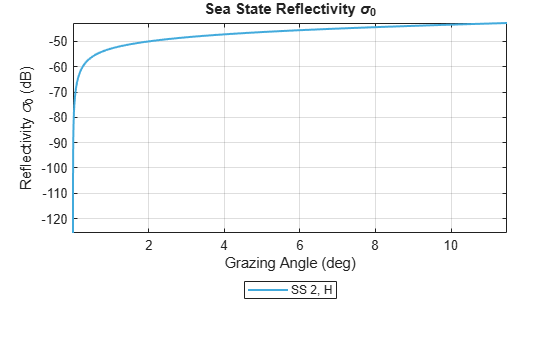Next, calculate the radar cross section (RCS) of the clutter using the clutterSurfaceRCS function. Note the drop in the clutter RCS as the radar horizon range is reached.

% Calculate clutter RCS rcs = clutterSurfaceRCS(nrcs,Rm,azbw,elbw,grazAng(:),tau); rcsdB = pow2db(rcs); % Convert to decibels for plotting hAxes = helperPlot(Rkm,rcsdB,'RCS','Clutter RCS (dBsm)','Clutter Radar Cross Section (RCS)'); helperAddHorizLine(hAxes,Rhoriz);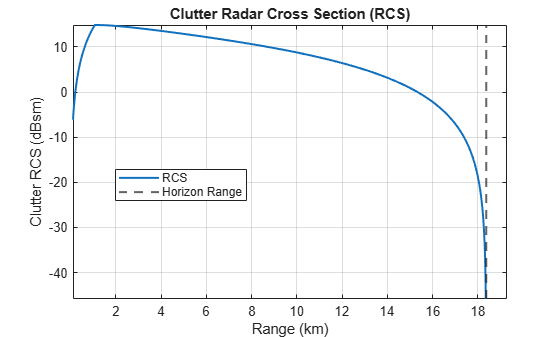Calculate the clutter-to-noise ratio (CNR) using the radareqsnr function. Again, note the drop in CNR as the simulation range approaches the radar horizon. Calculate the range at which the clutter falls below the noise.

% Convert frequency to wavelength lambda = freq2wavelen(freq); % Calculate and plot the clutter-to-noise ratio cnr = radareqsnr(lambda,Rm(:),ppow,tau,... 'gain',[Gt Gr],'rcs',rcs,'Ts',Ts); % dB hAxes = helperPlot(Rkm,cnr,'CNR','CNR (dB)','Clutter-to-Noise Ratio (CNR)'); ylim(hAxes,[-80 100]); helperAddHorizLine(hAxes,Rhoriz); helperAddBelowClutterPatch(hAxes);% Range when clutter falls below noise helperFindClutterBelowNoise(Rkm,cnr);
Range at which clutter falls below noise (km) = 18.04 

#### Considering the Propagation Path

When the path between the radar and clutter deviates from free space conditions, it is recommended to include the clutter propagation factor and the atmospheric losses on the path. The clutter propagation factor can be calculated using the radarpropfactor function.

% Calculate radar propagation factor for clutter Fc = radarpropfactor(Rm,freq,anht,surfht, ... 'SurfaceHeightStandardDeviation',hgtsd,... 'SurfaceSlope',beta0,... 'ElevationBeamwidth',elbw); helperPlot(Rkm,Fc,'Propagation Factor', ... 'Propagation Factor (dB)', ... 'One-Way Clutter Propagation Factor F_C');Within the above plot, 2 propagation regions are visible:

1. Interference region, where reflections interfere with the direct ray. This is exhibited over the ranges where there is lobing.

2. Intermediate region. This is the region between the interference and diffraction region, a shadow region beyond the horizon. The intermediate region, which in this example occurs at the kink in the curve at about 1.5 km, is generally estimated by an interpolation between the interference and diffraction regions.

Typically the clutter propagation factor and the sea reflectivity are combined as the product ${\sigma }_{\mathit{C}}{\mathit{F}}_{\mathit{C}\text{\hspace{0.17em}}}^{4}$, because measurements of surface reflectivity are generally measurements of the product rather than just the reflectivity ${\sigma }_{\mathit{C}}$. Calculate this product and plot the results.

% Combine clutter reflectivity and clutter propagation factor FcLinear = db2mag(Fc); % Convert to linear units combinedFactor = nrcs.*FcLinear.^2; combinedFactordB = pow2db(combinedFactor); helperPlot(Rkm,combinedFactordB,'\sigma_CF_C', ... '\sigma_CF_C (dB)', ... 'One-Way Sea Clutter Propagation Factor and Reflectivity');Next calculate the atmospheric loss on the path using the slant-path tropopl function. Use the default standard atmospheric model for the calculation.

% Calculate one-way loss associated with rain elAng = height2el(surfht,anht,Rm); % Elevation angle (deg) numEl = numel(elAng); Latmos = zeros(numEl,1); for ie = 1:numEl Latmos(ie,:) = tropopl(Rm(ie),freq,anht,elAng(ie)); end helperPlot(Rkm,Latmos,'Atmospheric Loss','Loss (dB)','One-Way Atmospheric Loss');Recalculate the CNR. Include the propagation factor and atmospheric loss in the calculation. Note the change in the shape of the CNR curve. The point at which the clutter falls below the noise is much closer in range when these factors are included.

% Re-calculate CNR including radar propagation factor cnr = radareqsnr(lambda,Rm(:),ppow,tau,... 'gain',[Gt Gr],'rcs',rcs,'Ts',Ts, ... 'PropagationFactor',Fc,... 'AtmosphericLoss',Latmos); % dB helperAddPlot(Rkm,cnr,'CNR + Propagation Factor + Atmospheric Loss',hAxes);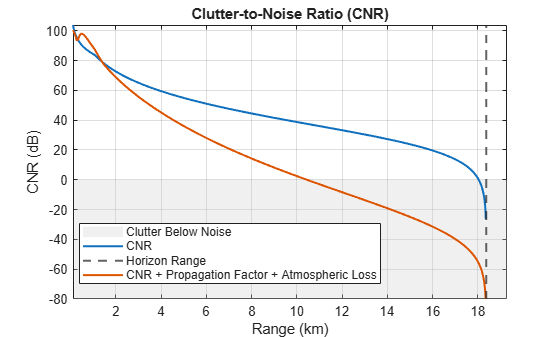% Range when clutter falls below noise helperFindClutterBelowNoise(Rkm,cnr);
Range at which clutter falls below noise (km) = 10.44 

#### Understanding Weather Effects

Just as weather affects the detection of a target, weather also affects the detection of clutter. Consider the effect of rain over the simulated ranges. First calculate the rain attenuation.

% Calculate one-way loss associated with rain rr = 50; % Rain rate (mm/h) polAng = 0; % Polarization tilt angle (0 degrees for horizontal) elAng = height2el(surfht,anht,Rm); % Elevation angle (deg) numEl = numel(elAng); Lrain = zeros(numEl,1); for ie = 1:numEl Lrain(ie,:) = cranerainpl(Rm(ie),freq,rr,elAng(ie),polAng); end helperPlot(Rkm,Lrain,'Rain Loss','Loss (dB)','One-Way Rain Loss');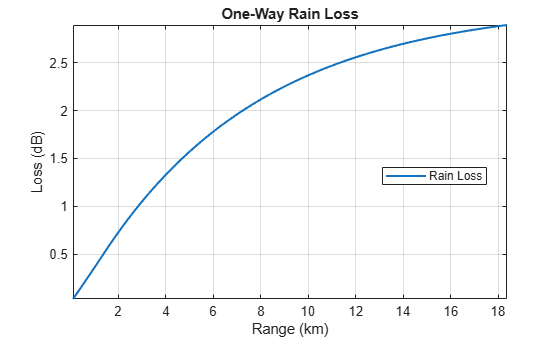Recalculate the CNR. Include the propagation path and the rain loss. Note that there is only a slight decrease in the CNR due to the presence of the rain.

% Re-calculate CNR including radar propagation factor and rain loss cnr = radareqsnr(lambda,Rm(:),ppow,tau,... 'gain',[Gt Gr],'rcs',rcs,'Ts',Ts, ... 'PropagationFactor',Fc, ... 'AtmosphericLoss',Latmos + Lrain); % dB helperAddPlot(Rkm,cnr,'CNR + Propagation Factor + Atmospheric Loss + Rain',hAxes);% Range when clutter falls below noise helperFindClutterBelowNoise(Rkm,cnr);
Range at which clutter falls below noise (km) = 9.61 

### Summary

This example introduced concepts regarding the simulation of sea surfaces. We learned that sea reflectivity exhibits the following properties:

• A strong dependence on sea state,

• A proportional dependence on frequency,

• A dependence on polarization that decreases with increasing frequency, and

• A strong dependence on grazing angle at low grazing angles

This example also discussed how to use the sea state physical properties and reflectivity for the calculation of the clutter-to-noise ratio for a maritime surveillance radar system. Additionally, the example explained ways to improve simulation of the propagation path.

### References

1. Barton, David K. Radar Equations for Modern Radar. 1st edition. Norwood, MA: Artech House, 2013.

2. Blake, L.V. Machine Plotting of Radar Vertical-Plane Coverage Diagrams. Naval Research Laboratory Report 7098, 1970.

3. Gregers-Hansen, V. and Mittal, R. "An Improved Empirical Model for Radar Sea Clutter Reflectivity." NRL/MR/5310-12-9346, Apr. 27, 2012.

4. Richards, M. A., Jim Scheer, and William A. Holm. Principles of Modern Radar. Raleigh, NC: SciTech Pub., 2010.

function helperPlotSeaRoughness(ss,hgtsd,beta0,vw) % Creates 3x1 plot of sea roughness outputs % Create figure figure % Plot standard deviation of sea wave height subplot(3,1,1) plot(ss,hgtsd,'-o','LineWidth',1.5) ylabel([sprintf('Wave\nHeight') '\sigma (m)']) title('Sea Wave Roughness') grid on; % Plot sea wave slope subplot(3,1,2) plot(ss,beta0,'-o','LineWidth',1.5) ylabel(sprintf('Wave\nSlope (deg)')) grid on; % Plot wind velocity subplot(3,1,3) plot(ss,vw,'-o','LineWidth',1.5) xlabel('Sea State') ylabel(sprintf('Wind\nVelocity (m/s)')) grid on; end function hAxes = helperPlotSeaReflectivity(ss,grazAng,freq,nrcs,pol,hAxes) % Plot sea reflectivities % Create figure and new axes if axes are not passed in newFigure = false; if nargin < 6 figure(); hAxes = gca; newFigure = true; end % Get polarization string switch lower(pol) case 'h' lineStyle = '-'; otherwise lineStyle = '--'; end % Plot if numel(grazAng) == 1 hLine = semilogx(hAxes,freq(:).*1e-9,pow2db(nrcs),lineStyle,'LineWidth',1.5); xlabel('Frequency (GHz)') else hLine = plot(hAxes,grazAng(:),pow2db(nrcs),lineStyle,'LineWidth',1.5); xlabel('Grazing Angle (deg)') end % Set display names numLines = size(nrcs,2); for ii = 1:numLines hLine(ii).DisplayName = sprintf('SS %d, %s',ss(ii),pol); if newFigure hLine(ii).Color = brighten(hLine(ii).Color,0.5); end end % Update labels and axes ylabel('Reflectivity \sigma_0 (dB)') title('Sea State Reflectivity \sigma_0') grid on axis tight hold on; % Add legend legend('Location','southoutside','NumColumns',5,'Orientation','Horizontal'); end function varargout = helperPlot(Rkm,y,displayName,ylabelStr,titleName) % Used in CNR analysis % Create figure hFig = figure; hAxes = axes(hFig); % Plot plot(hAxes,Rkm,y,'LineWidth',1.5,'DisplayName',displayName); grid(hAxes,'on'); hold(hAxes,'on'); xlabel(hAxes,'Range (km)') ylabel(hAxes,ylabelStr); title(hAxes,titleName); axis(hAxes,'tight'); % Add legend legend(hAxes,'Location','Best') % Output axes if nargout ~= 0 varargout{1} = hAxes; end end function helperAddPlot(Rkm,y,displayName,hAxes) % Used in CNR analysis % Plot ylimsIn = get(hAxes,'Ylim'); plot(hAxes,Rkm,y,'LineWidth',1.5,'DisplayName',displayName); axis(hAxes,'tight'); ylimsNew = get(hAxes,'Ylim'); set(hAxes,'Ylim',[ylimsIn(1) ylimsNew(2)]); end function helperAddHorizLine(hAxes,Rhoriz) % Add vertical line indication horizon range xline(Rhoriz.*1e-3,'--','DisplayName','Horizon Range','LineWidth',1.5); xlims = get(hAxes,'XLim'); xlim([xlims(1) Rhoriz.*1e-3*(1.05)]); end function helperAddBelowClutterPatch(hAxes) % Add patch indicating when clutter falls below the noise xlims = get(hAxes,'Xlim'); ylims = get(hAxes,'Ylim'); x = [xlims(1) xlims(1) xlims(2) xlims(2) xlims(1)]; y = [ylims(1) 0 0 ylims(1) ylims(1)]; hP = patch(hAxes,x,y,[0.8 0.8 0.8], ... 'FaceAlpha',0.3,'EdgeColor','none','DisplayName','Clutter Below Noise'); uistack(hP,'bottom'); end function helperFindClutterBelowNoise(Rkm,cnr) % Find the point at which the clutter falls below the noise idxNotNegInf = ~isinf(cnr); Rclutterbelow = interp1(cnr(idxNotNegInf),Rkm(idxNotNegInf),0); fprintf('Range at which clutter falls below noise (km) = %.2f\n',Rclutterbelow) end